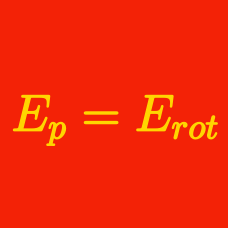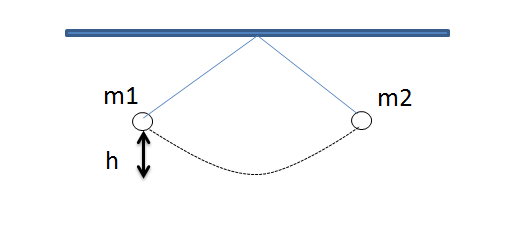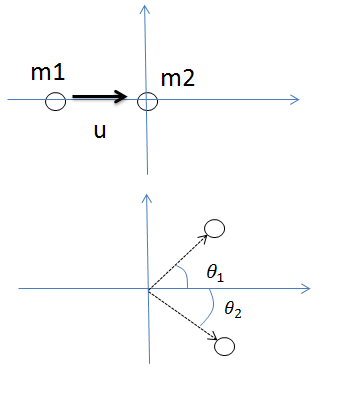Classical Mechanics

# Elastic collisions

A body of mass $m = 5 \text{ kg}$ collides elastically with a stationary mass $M = 9 \text{ kg}$ on the $x$-axis. Before the collision, the velocity of mass $m$ is $+2 \text{ m/s}$. If the velocity of mass $M$ after the collision is $v_2 = \frac{a}{b}\text{ m/s},$ where $a$ and $b$ are coprime positive integers, what is the value of $a+b?$Two pendulums with respective masses of $m_1 = 5 \text{ kg}$ and $m_2 = 10 \text{ kg}$ hang from the same point by identical strings, as shown in the figure above. Both being initially $h = 1 \text{ m}$ high from the lowest point, they swing down and collide elastically at the lowest point. If the velocity of mass $m_1$ after the collision $v_1$ satisfies $v_1^2=\frac{a}{b},$ where $a$ and $b$ are coprime positive integers, what is the value of $a+b?$A body of mass $m_1 = 5 \text{ kg}$ collides elastically with a stationary mass $m_2 = 4 \text{ kg}.$ Before the collision, the velocity of mass $m_1$ is $u = 2 \text{m/s} .$ After collision, masses $m_1$ and $m_2$ move in directions that make respective angles of $\theta_1$ and $\theta_2$ with the original direction that $m_1$ had moved in. If $\sin{\theta_1} = \frac{4}{5}$ , what is the velocity of mass $m_1$ after the collision?

Consider a one dimensional collision between two point masses with different masses $m_1$ and $m_2$ and initial velocities $v_1$ and $v_2$. We define the ratio $r$ as the ratio of the difference in initial velocities to the difference in final velocities:

$r=\frac{|v_{1,i}-v_{2,i}|} {|v_{1,f}-v_{2,f}|}$.

What is $r$ if the collision is perfectly elastic?Two pendulums with respective masses of $m_1 = 4 \text{ kg}$ and $m_2 = 8 \text{ kg}$ hang from the same point by identical strings, as shown in the figure above. Both being initially $h = 18 \text{ m}$ high from the lowest point, they swing down and collide elastically at the lowest point. What is the maximum height that pendulum $m_1$ reaches after the collision?

×